#Cartesian Coordinates

Pre-requisites: N-Tuples.

There are many ways to study and describe geometric objects in three-dimensional space. One method, pioneered by the Greeks and formalized by Euclid, is to study these objects axiomatically: to define points, lines, planes and other such entities by the axioms they satisfy ("every two distinct points define a line", for instance).

Another method invented by Descartes [ Oresme actually thought of it first, but never gets any credit] reduces geometry to algebra through the following procedure:

• First, pick some point O as the origin, and then draw three mutually perpendicular lines through the origin. These lines are labeled as the x-axis, the y-axis and the z-axis, and all together are refered to as the coordinate axes.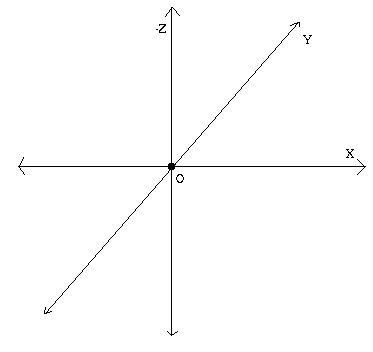(In the above figure, and the one below, the y axis is pointing into the page.)

• Next, give each of these axes a positive side and a negative side. There are 23 = 8 ways of doing this, but you can always rotate and the set of axes so that there end up being only only two configurations: a right-handed one, and a left-handed one. By a right-handed configuration, we mean the axes system you get by sticking out the thumb, pointer finger, and middle finger of your right hand so that they are perpendicular to each other, and then calling your thumb the positive x-axis, your pointer finger the positive y-axis, and your middle finger the positive z-axis. Try as you may, you will never be able to rotate these axes onto the axes system you get by doing the same procedure with your left hand.

You will almost always want to be using a right handed axis system. Exceptions are so rare as to be negligible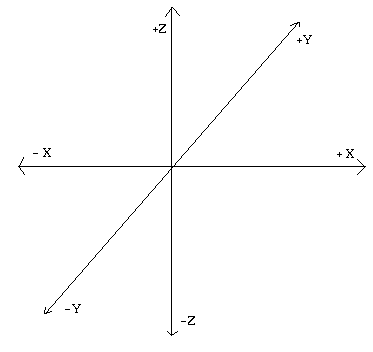• Now that we have an oriented coordinate axis system, we can convert points to triplets simply by projecting the point onto each of our axes. If my point P projects onto the positive x axis at a distance of 4 units away from the origin, and onto the negative y axis at a distance of 5 units away from the origin, and onto the negative z axis at a distance of 3 units away from the origin, then we get the triplet (4,-5,-3) as representing the position of the point P. These numbers are called the cartesian coordinates of P, and every point has a unique triplet of cartesian coordinates.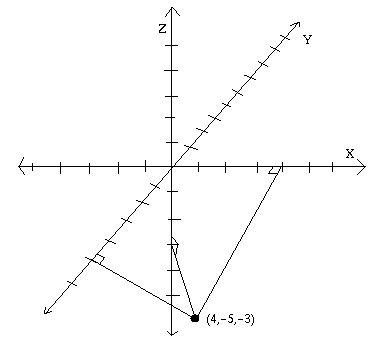• Similarly, every set of cartesian coordinates determines a point. To get the point with coordinates (2,3.5,-1.03), simply start at the origin, go 2 units along the positive x axis, draw a line there parallel to the y axis, go 3.5 units along this line in the positive y-direction, then draw a line parallel to the z axis and go 1.03 units along it in the negative z-direction. You are now at the desired point.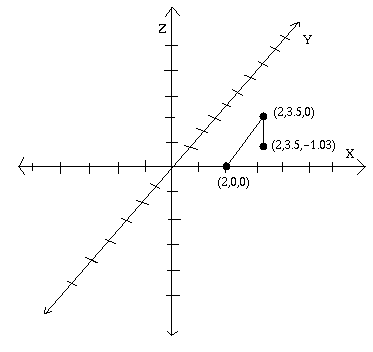• The above procedure worked for points in three dimensions; you could also drop the z axis to get cartesian coordinates in the plane. "The plane" always refers to the x-y plane, the plane containing the x and y axes. Similarly, the x-z plane is the plane containing the yx and z axes, and the y-z plane is the plane spanned by the y and z axes.

It would be hard to overstate the importance of Cartesian coordinates in the history (and practice) of mathematics. Previously unsolvable geometric problems could be converted to long, tedious, but doable calculations in algebra. [Contrast with the Greek era when even simple algebraic formulas were converted into cumbersome geometric figures.] The whole field of trigonometry makes the most sense once you've introduced Cartesian axes. And, much insight is gained into a complicated algebraic formula when the formula can be graphed, which after all is nothing more than drawing all the points with cartesian coordinates of the form (xf(x)). Or, as we will do shortly, of the form (xyf(xy)).

### Exercises:

1. Draw a right-handed and a left-handed coordinate axis system. Be sure to label your axes and to indicate the positive and negative directions on each one.

2. What are the cartesian coordinates of the origin? What can you say about the cartesian coordinates of a point on the x axis? Of a point in the y-z plane?

3. Draw a coordinate axis system, and then find and label the following points: (0,6,0), (3,0,-1), (1,2,3).

4. Prove the three-dimensional Pythagorean theorem: If a point P has coordinates (x1x2x3), then its distance from the origin is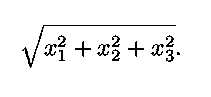You may assume the normal two-dimensional Pythagoren theorem.

Solutions to Exercises | Vector Calculus Index | World Web Math Categories Page

watko@athena.mit.edu
Last modified November 20, 1998